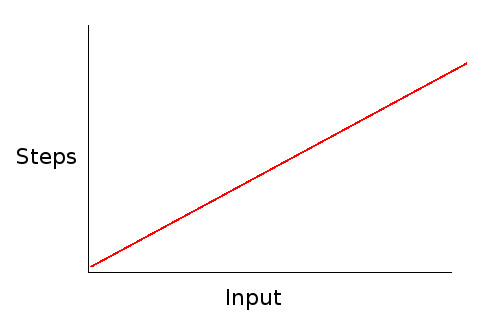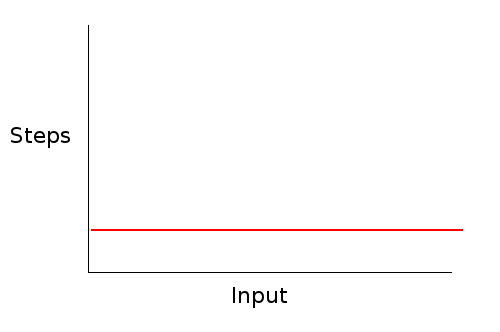# Algorithm Analysis

### Measuring Algorithm Speed

There are usually many algorithms that solve the same problem. Oftentimes some of these algorithms are more efficient than others.

As we've discussed, the choice between different data structures also has a big effect on the efficiency of programs. For example, removing something from a linked list is a lot faster than removing something from an array. Likewise, adding to the end of a doubly-linked list is a lot faster than adding to the end of a singly-linked list.

But how much faster are these things? Today we'll look at ways of measuring how efficient different algorithms and data structures are.

### Using Execution Time

One way of measuring how fast an algorithm is is to actually time it. We can write multiple programs, compile them, and run them to see which one runs faster.

If we try running these programs, we'll see the affect of using a singly vs. doubly linked list. If we only add a few elements, the program is so fast that it makes little difference. Storing a lot of data, however, and the difference becomes clear:

 Number of Elements Singly-Linked Doubly-Linked 100 .004 .004 1,000 0.01 s 0.004 s 10,000 0.28 s 0.007 s 50,000 7.5 s 0.013 s 100,000 30.6 s 0.019 s 200,000 2m 16s 0.036 s

As you can see, the difference is enormous, the singly linked list takes over 3,000 times longer to add 200,000 nodes to the end of the list.

Timing programs like this gives a very clear idea of how efficient a given program is. But there are some drawbacks:

• It only can really compare two algorithms. Timing only one program doesn't give you an objective measure of its speed.
• There is a large variation in the time it takes to run a program.
• It only gives you a measure of a whole program - not individual functions or algorithms.
• It forces you to test multiple input sizes. If we only tested up to a thousand, there would have been little difference.

### Order of Growth

An alternative way to quantify the efficiency of an algorithm is to express it in terms how much more work we have to do for larger and larger inputs. The algorithm to add a node at the end of a singly-linked list is given below:

1. Create the new node with the right data.
2. Set the new node's next field to NULL.
3. If the list is empty, set the head to the new node and return.
4. Search through the list from the head for the last node:
1. Check if this node is last.
2. If not, move onto the next node.
5. Set the last node's next field to the new node.

If we have an empty list, this algorithm will not need to loop at all looking for the end, so the algorithm will have 4 steps. If we have 10 items, the algorithm will loop through all 10 items to find the end, and have 24 steps (20 for the loop and 4 for the others). If we have 100 items, the algorithm will loop through all 100 to find the end, and have 204 steps. With 1000 items, the algorithm will have 2004 steps.

When we start having more and more nodes, it should be clear that the loop dominates the algorithm. In algorithm analysis, we are just looking for a rough idea of how many steps an algorithm takes. The fact that there are 4 extra steps doesn't really matter.

Likewise, the fact that there are two steps in the loop doesn't really matter. When we turn this algorithm into a program, the loop could have more or less C++ instructions. Likewise, the compiler can turn a C++ instruction into any number of machine instructions.

What we are really interested in is how the number of steps scales as we have larger and larger input. This algorithm scales linearly because the number of steps is a linear function of the input size:### Big-O Notation

Big-O notation is how algorithm efficiency is expressed. It basically expresses how the runtime of an algorithm scales with the input. In Big-O notation, the algorithm above is expressed as $O(n)$.

The $O$ stands for "order of" and the $n$ is how big the input to the algorithm is. So with an input size of $n$ (the number of things in the linked list), this algorithm take around $n$ steps to complete.

The algorithm for adding to the end of a doubly linked list is given below:

1. Make the new node with the given data.
2. Set the node's next to NULL.
3. If the list is empty:
1. Set the head to node.
2. Set the tail to node.
3. Set node's prev to NULL.
4. Otherwise:
1. Set node's prev to tail.
2. Set tail's next to node.
3. Set tail to node.

There is no loop in this algorithm. It will always take the same number of steps no matter how big or small the list is. This means that the algorithm is constant time. This is expressed as $O(1)$ in Big-O notation.### Definition of Big-O

If $f(n)$ and $g(n)$ are functions, then:

$f(n) = O(g(n))$

Is true if there is some constant value $c$ that we can multiply $g(n)$ and have $g(n)$ be greater than or equal to $f(n)$ for sufficiently large values of $n$.

Big-O basically lets us simplify a function. For example, if:

$f(n) = 2*n + 4$

Then we can say that:

$f(n) = O(n)$

Because there is some value $c$ (for example 3) where, for sufficiently large values of $n$, $3 \cdot n$ is greater than $2 \cdot n + 4$.

Big-O analysis basically only considers the "order" of a function such as whether it is constant, linear, quadratic or exponential, rather than the full definition of the function.

If an algorithm has an efficiency of $O(n)$ it means that, as the size of the input increases, the running time of the algorithm increases in a linear way. Adding to the end of a singly-linked list is a $O(n)$ operation.

A complexity of $O(1)$ means that an algorithm takes a constant amount of time to run no matter how big the input gets. Adding to the end of a doubly-linked list is a $O(1)$ operation.

The smaller the complexity, the better. Note, however, that for small values of $n$, a $O(n)$ algorithm could actually run faster. For example, adding to an empty singly-linked list could be faster than adding to an empty doubly-linked list. However, what happens with larger input sizes is generally all we care about.

### Finding the Order of an Algorithm

To find the complexity of an algorithm, you need to examine it, and see how it will execute with larger sized inputs. If there is a loop that goes up to the size of the input, it will be at least $O(n).$ With nested loops, the maximum loop values are multiplied.

What is the Big-O complexity of the following function?


int max(int* array, int size) {
int max_so_far = array;

for(int i = 1; i < size; i++) {
if(max_so_far < array[i]) {
max_so_far = array[i];
}
}
}


void printMultTable(int size) {
for(int i = 0; i <= size; i++) {
for(int j = 0; j <= size; j++) {
cout << i * j << " ";
}
cout << endl;
}
}

1. Make a new, resized array.
2. Copy the elements of the old array into the new one.
3. De-allocate the old array.
4. Reset the old array to point at the new one.
This algorithm to push onto an array stack?

// push on a new value
void push(char item) {
top++;
array[top] = item;
}

We will see many more examples of algorithm analysis through the semester!